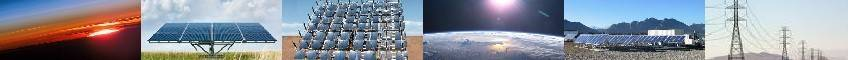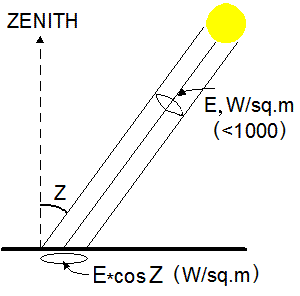# THE BASIC FACTS and INFORMATION ABOUT SOLAR ENERGY## WHAT IS IT?

The sun is shining brightly every day, bathing the world with light and heat. But besides for these benefits, sunlight also miraculously carries energy, which can be captured and used for a variety of purposes. Before we go over practical solar energy facts, let's first quickly revisit sunlight origin and properties. The nuclear reactions within the Sun produce among other things electromagnetic radiation which is emitted into space. It has a dual nature as both particles and waves, which can transport energy through empty space. This is what's casually called solar energy. When it hits material objects, it can naturally convert directly into three usable forms of energy: electric, thermal, and chemical. It can also convert indirecty into other forms, such as wind. This page will deal only with conversion of sunlight into electricity.

## HOW MUCH SUNLIGHT DO WE GET?

The scientists estimate the net amount of power emitted from the Sun is approximately 63 MW for each square meter of its surface. Its intensity drops with the squared distance from the Sun as the sphere of this emission is expanding. From geometrical concepts we can find that an average density at the top Earth's atmosphere is about 46,000 times less. Just for a reference, there is some dispute about its exact value. According to National Institute of Standards and Technology (NIST), the currently accepted value is 1366 watt/sq.m, while according to NASA's SORCE measurements it is 1361. If we take into account the fact that only half the Earth is lit at one time and if we divide the total amount of the irradiance intercepted by the Earth by its surface area, after simple math we'll get 1/4 of the above quantity, or approximately 341 watts/sq. meter. Only about half of this amount actually reaches our planet's surface. The above number has only a theoretical interest and is useless for all practical purposes because the sunlight, of course, does not fall on the entire planet evenly. Therefore, let's talk about more useful numbers. What I am going to tell you may sound a bit too technical, but this is what you might want to know if you are considering buying a home PV system and want to know exactly how much electricity it can produce for you.

Sunlight intensity obviously varies by time and latitude. Not surprisingly, the irradiance is largest near the equator. Numerically, on a clear day at noon at sea level it is close to 1000W per meter squared. The solar industry casually refers to this value as "standard sun".## PRACTICAL NUMBERS ON SOLAR ELECTRICITY

Here is a quick summary of the main facts, definitions and equations related to solar panels and electricity generation.
• Irradiance (average power measured at the top Earths atmosphere perpendicular to the sunrays): 1,366 watt per square meter;
• Peak solar power flux at noon on a perpendicular surface at sea level: 1 kW/sq.m (remember 1 kilowatt is 1000 watts);
• Insolation (average net daily sunlight per square meter) at optimum panels tilt: 4 to 7 kWh depending on your location;
• Total electric energy produced by a PV array over a day: Epv=Insolation×Efficiency/100 kWh/m2, where panel's efficiency is from 6% to 22%.

You can use the above data to calculate practically everything else. Here are some examples of the results:
• For the cells with 20% efficiency, the daily yield would be Epv=1000W/m2×(4...7)×0.2=800 to 1400 watt-hours per m2.
• Solar power generated by PV panels averaged over a day: Ppv=Epv/24. For the most efficient models this yields 33 to 58 watt/m2.
Note that the above numbers are related just to DC output of PV panels at 25oC. In a complete system there will be additional energy losses of 3 to 10% in the inverter and another 3-5% in the wiring. In addition, PV cell efficiency drops as it heats up. So, in reality the resulting net amount of solar energy may be 10 to 20% lower.
• Net solar electricity generated over a day by a PV system per each kW of nameplate DC power: ≈0.8×Insolation, where 0.8 is a factor that accounts for losses in inverter and wires. For example, in the area with insolation 5 kWh/m2 a 5,000 watt system will produce 5x5x0.8=20 kWh over a day, which is just 20/24=833 watt in average.

Note that 1 ft^2=0.0929 m^2. So, if you want to get the numbers per square foot, roughly, they will be 10 times less than the respective numbers per square meter.

## QUICK REFERENCE INFORMATION ABOUT SUN

The Sun just like probably all stars, consists mainly of hydrogen and heliumis. High pressure and temperature in the Sun's core cause hydrogen atoms to break up. Their nuclei then combine forming helium nuclei. This process is called nuclear fusion. In this reaction, the resulting atoms have less internal energy than the starting particles. Since energy is conserved, the balance of it is released in the form of heat, photons and other particles. The photons are constantly intercepted, absorbed and re-emitted by surrounding molecules. Eventually they reach the surface and emitted to outer space. As we metioned above, this emission is referred to as radiated solar energy.

In conclusion, below is a brief summary of information about the Sun.
Diameter: 1,392,000 km (863,040 miles);
Mass: 1,989,100×1024 kg;
Outside temperature: ~5700 oC;
Average Earth-Sun Distance: 150 million km (93 million miles);
Content by mass: 74% Hydrogen, 25% Helium, 1% other;
Luminosity (total amount of power radiated in all directions): 3.85×1026 watt (~385 billion megawatts);
Radiated power density at Sun's surface: 63,300 kW/m2.